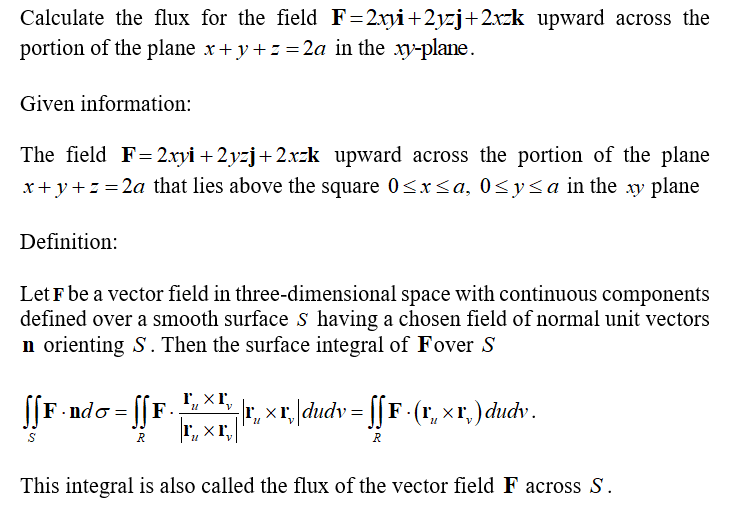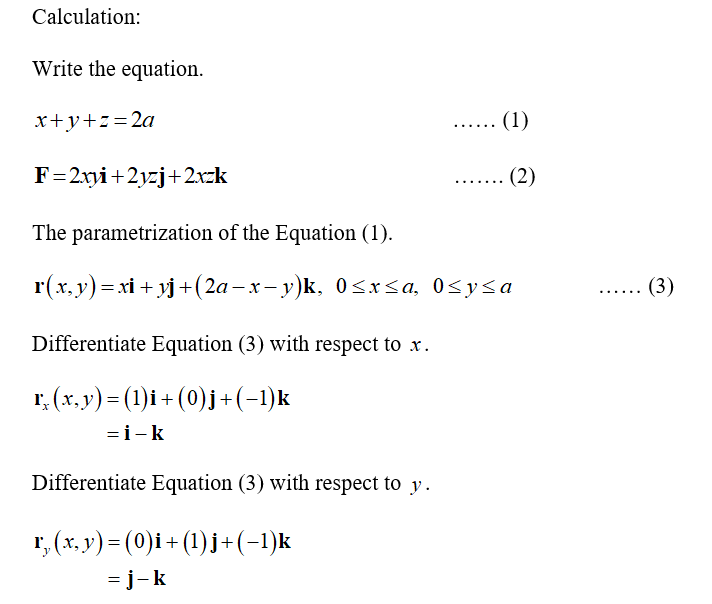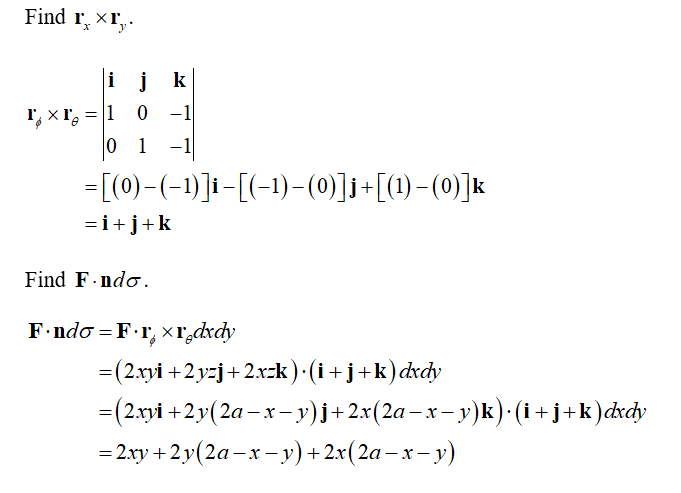# Find the flux of the field F = 2xy i + 2yz j + 2xz k upward across the portion of the plane x + y + z = 2a that lies above the square 0 <=x <=a, 0<= y <=a, in the xy-plane.

Question
1 views

Find the flux of the field F = 2xy i + 2yz j + 2xz k upward across the portion of the plane x + y + z = 2a that lies above the square 0 <=x <=a, 0<= y <=a, in the xy-plane.

check_circle

Step 1Step 2Step 3...

### Want to see the full answer?

See Solution

#### Want to see this answer and more?

Solutions are written by subject experts who are available 24/7. Questions are typically answered within 1 hour.*

See Solution
*Response times may vary by subject and question.
Tagged in